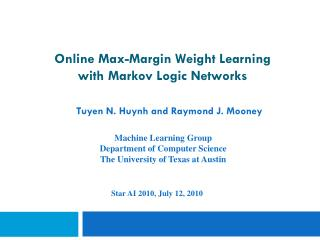# Online Max-Margin Weight Learning with Markov Logic Networks - PowerPoint PPT PresentationDownload PresentationOnline Max-Margin Weight Learning with Markov Logic Networks

Online Max-Margin Weight Learning with Markov Logic NetworksDownload Presentation## Online Max-Margin Weight Learning with Markov Logic Networks

- - - - - - - - - - - - - - - - - - - - - - - - - - - E N D - - - - - - - - - - - - - - - - - - - - - - - - - - -
##### Presentation Transcript

1. Machine Learning Group Department of Computer Science The University of Texas at Austin Online Max-Margin Weight Learning with Markov Logic Networks Star AI 2010, July 12, 2010 Tuyen N. Huynh and Raymond J. Mooney

2. Outline • Motivation • Background • Markov Logic Networks • Primal-dual framework • New online learning algorithm for structured prediction • Experiments • Citation segmentation • Search query disambiguation • Conclusion

3. Motivation • Most of the existing weight learning for MLNs are in the batch setting. • Need to run inference over all the training examples in each iteration • Usually take a few hundred iterations to converge • Cannot fit all the training examples in the memory  Conventional solution: online learning

4. Background

5. Markov Logic Networks (MLNs) [Richardson & Domingos, 2006] • An MLN is a weighted set of first-order formulas • Larger weight indicates stronger belief that the clause should hold • Probability of a possible world (a truth assignment to all ground atoms) x: 2.5Center(i,c) => InField(Ftitle,i,c) 1.2InField(f,i,c) ^ Next(j,i) ^ ¬HasPunc(c,i)=> InField(f,j,c) Weight of formula i No. of true groundings of formula i in x

6. Existing discriminative weight learning methods for MLNs • maximize the Conditional Log Likelihood (CLL)[Singla & Domingos, 2005], [Lowd & Domingos, 2007], [Huynh & Mooney, 2008] • maximize the margin, the log ratio between the probability of the correct label and the closest incorrect one [Huynh & Mooney, 2009]

7. Online learning

8. Primal-dual framework [Shalev-Shwartzet al., 2006] • A general and latest framework for deriving low-regret online algorithms • Rewriting the regret bound as an optimization problem (called the primal problem), then considering the dual problem of the primal one • A condition that guarantees the increase in the dual objective in each step  Incremental-Dual-Ascent (IDA) algorithms. For example: subgradient methods

9. Primal-dual framework (cont.) • Proposed a new class of IDA algorithms called Coordinate-Dual-Ascent (CDA) algorithm: • The CDA update rule only optimizes the dual w.r.t the last dual variable • A closed-form solution of CDA update rule  CDA algorithms have the same cost as subgradient methods but increase the dual objective more in each step  converging to the optimal value faster

10. Primal-dual framework (cont.)

11. CDA algorithms for max-margin structured prediction

12. Max-margin structured prediction

13. Steps for deriving new CDA algorithms • Define the regularization and loss functions • Find the conjugate functions • Derive a closed-form solution for the CDA update rule

14. 1. Define the regularization and loss functions Label loss function

15. 1. Define the regularization and loss functions (cont.)

16. 2. Find the conjugate functions

17. 2. Find the conjugate functions (cont.)

18. 3. Closed-form solution for the CDA update rule • Optimization problem: • Solution:

19. CDA algorithms for max-margin structured prediction

20. Experiments

21. Citation segmentation • Citeseer dataset [Lawrence et.al., 1999] [Poon and Domingos, 2007] • 1,563 citations, divided into 4 research topics • Each citation is segmented into 3 fields: Author, Title, Venue • Used the simplest MLN in [Poon and Domingos, 2007] • Similar to a linear chain CRF: Next(j,i) ^ !HasPunc(c,i) ^ InField(c,+f,i) => InField(c,+f,j)

22. Experimental setup • Systems compared: • MM: the max-margin weight learner for MLNs in batch setting [Huynh & Mooney, 2009] • 1-best MIRA [Crammer et al., 2005] • Subgradient [Ratliff et al., 2007] • CDA1/PA1 • CDA2

23. Experimental setup (cont.) • 4-fold cross-validation • Metric: • CiteSeer: micro-average F1 at the token level • Used exact MPE inference (Integer Linear Programming) for all online algorithms and approximate MPE inference (LP-relaxation) for the batch one. • Used Hamming loss as the label loss function

24. Average F1

25. Average training time in minutes

26. Microsoft web search query dataset • Used the clean-up dataset created by Mihalkova & Mooney  • Has thousands of search sessions where an ambiguous queries was asked • Goal: disambiguate search query based on previous related search sessions • Used 3 MLNs proposed in [Mihalkova & Mooney, 2009]

27. Experimental setup • Systems compared: • Contrastive Divergence (CD) [Hinton 2002]: used in [Mihalkova & Mooney, 2009] • 1-best MIRA • Subgradient • CDA1/PA1 • CDA2 • Metric: • Mean Average Precision (MAP): how close the relevant results are to the top of the rankings

28. MAP scores

29. Conclusion • Derived CDA algorithms for max-margin structured prediction • Have same computational cost as existing online algorithms but increase the dual objective more • Experimental results on two real-world problems show that the new algorithms generally achieve better accuracy and also have more consistent performance.

30. Thank you! Questions?